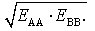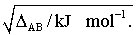# How does the electronegativity scale work

## Electronegativity

Electronegativity, a relative measure of the tendency of an atom in a molecule to attract binding electron pairs. The E. is an important criterion for estimating the polarity of a bond, which was developed by L. Pauling (1932). It was assumed that the binding energy E.FROM for a heteronuclear compound AB is always greater than the arithmetic or geometric mean value of the binding energies of AA and BB: E.FROM > 1/2(E.AA + E.BB) or.

E.FROM >This indicates that the heteronuclear molecule is stabilized with respect to the homonuclear molecules by an additional electrostatic contribution. The additional stabilization energy ΔFROM (in kJ mol-1) should therefore be a measure of the polarity of the bond. Using the geometric mean one obtains:

ΔFROM = E.FROMUsing ΔFROM can the

Difference of E. of atoms A and B can be defined:

| χA. - χB.| = 0,088By arbitrarily assigning a value χ for the element fluorineF. = 4, a closed electronegativity scale can be specified for the elements on the basis of the electronegativity differences (table). It can be seen that the E. represents a periodic property of the elements. The E. cannot be measured and is given as a dimensionless number. Mulliken (1934) was able to show that the sums from the 1st ionization energy E.I. and electron affinity E.A. (both values ​​in kJ mol-1) are proportional to the electronegativity values ​​according to Pauling to a very good approximation. In this way a numerically similar electronegativity scale can be given: χ = (E.A. + E.I.) / 523.3 kJ mol-1. In addition, other electronegativity scales with similar numerical values ​​were set up using different quantities. The E. is not an atomic constant, but depends to a large extent on the bonding state of the atom and on its neighboring atoms in the molecule. On this basis, taking into account the various electronegativity scales of Bažzanov (1962) presented a system of electronegativity values. All electronegativity scales have in common that the polarity and with it the Ionic character the bond becomes stronger as the difference in electronegativity of the atoms involved increases.

Electronegativity. Tab .: Electronegativity values ​​of some main group elements (according to Pauling).